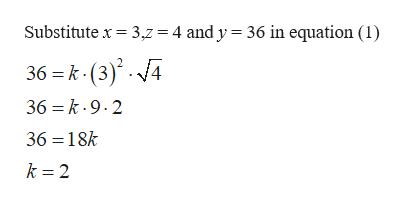# Use the given information to find the unknown value.y varies jointly as the square of x and the square root of z. Whenx = 3andz = 4,theny = 36.Find y whenx = 9andz = 36.

Question

Use the given information to find the unknown value.

y varies jointly as the square of x and the square root of z. When
x = 3
and
z = 4,
then
y = 36.

Find y when

x = 9

and

z = 36.
check_circleExpert Solution
Step 1

Given,

y varies jointly as the square of x and the square root of z.

Step 2

To find k:

...help_outlineImage TranscriptioncloseSubstitute x 3,z = 4 and y = 36 in equation (1) 36 k (3).4 36 k.9.2 36 18k k 2 fullscreen

### Want to see the full answer?

See Solution

#### Want to see this answer and more?

Solutions are written by subject experts who are available 24/7. Questions are typically answered within 1 hour*

See Solution
*Response times may vary by subject and question
Tagged in

### Equations and In-equations